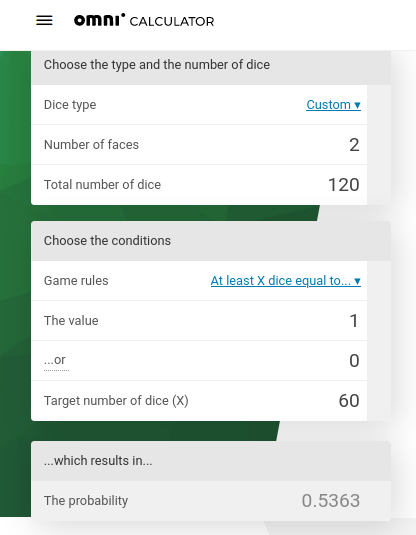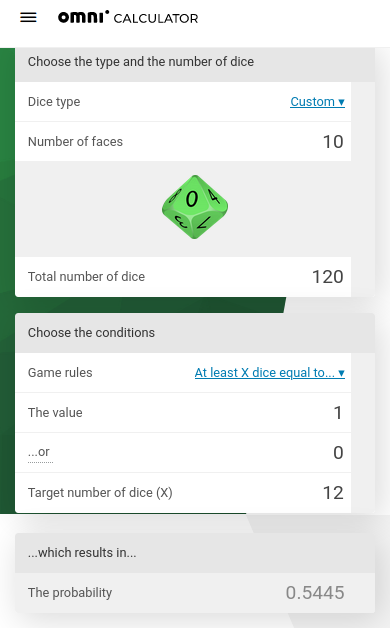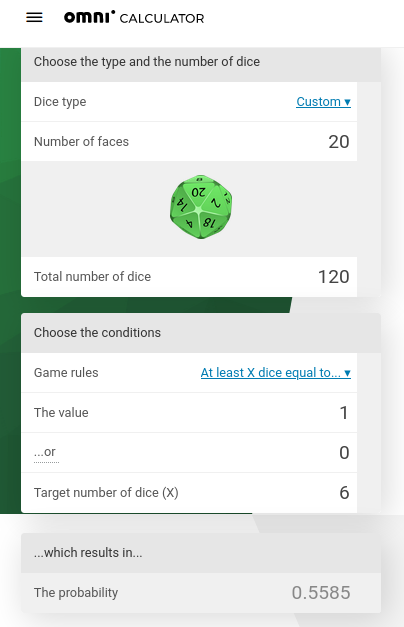# The Randomness of Mining

The PoX consensus algorithm says that the probability to win the sortition for the next block is the committed BTC amount divided by the total committed BTC amount by all miners.

If there are two miners,

• each committing the same BTC amount then the probability to get the block reward is 50:50.
• one committing twice as much as the first one, then the probability is 66:33. Note, the first miner doubled the commitment but the probability only increased by 16%.

What is the probability to get at least the portion of rewards related to the committed Bitcoins?

Example: Two miners, each committing the same amount of sats for 120 blocks, then the probability to get at half of the total rewards of 240,000 STX or more is *53,63% (according to Dice Probability Calculator):Similar for 10 or 20 miners that all pay the same amount of BTC:So don’t expect to get the rewards proportional to your btc spending early on. Law of big numbers kicks in only later.

Do you have more questions like this?

1 Like